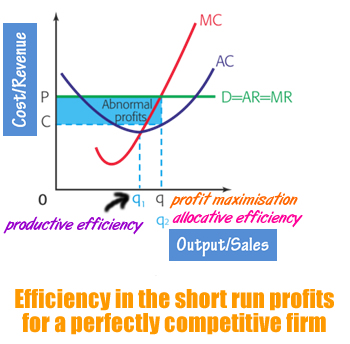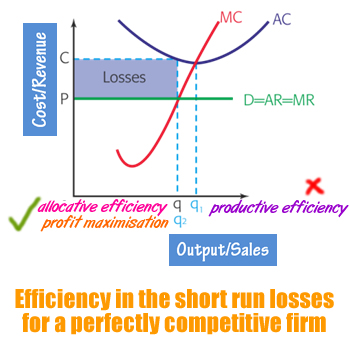# Efficiency

## In the long run

A perfectly competitive market will have both productive efficiency and allocative efficiency in the long run.### Productive efficiency

As we know productive efficiency level of production is where MC=AC . That means is known to be productively efficient if it is producing at a point where MC=AC, because MC always cuts AC at its lowest point.
In the case of Perfect Competition, a firm produces at productive efficient level of output q as shown in the diagram

### Allocative efficiency

This is the socially optimal level of output. At this point it is impossible to make one person better off without making someone else worse. There is pareto optimality.
It occurs where MC = AR
In other words, a firm in a perfectly competitive market produces at the profit maximising level which is MR=AR. This is also the point where MC=AR. Thus we conclude that in perfect competition there is allocative efficiency in the long run.

## In the Short run

In the short run, a firm in the perfectly competitive market may not achieve allocative efficiency and productive efficiency.

### When a firm is making abnormal profit

The firm produces at q which is both profit maximising level [MC=MR ] and also the allocative efficient level q2 [MC=AR]. However there is no productive efficiency q1.### When a firm is making abnormal loss

The firm produces at q which is both profit maximising level [MC=MR] and also the allocative efficient level q2 [MC=AR]. However there is not productive efficiency q1.